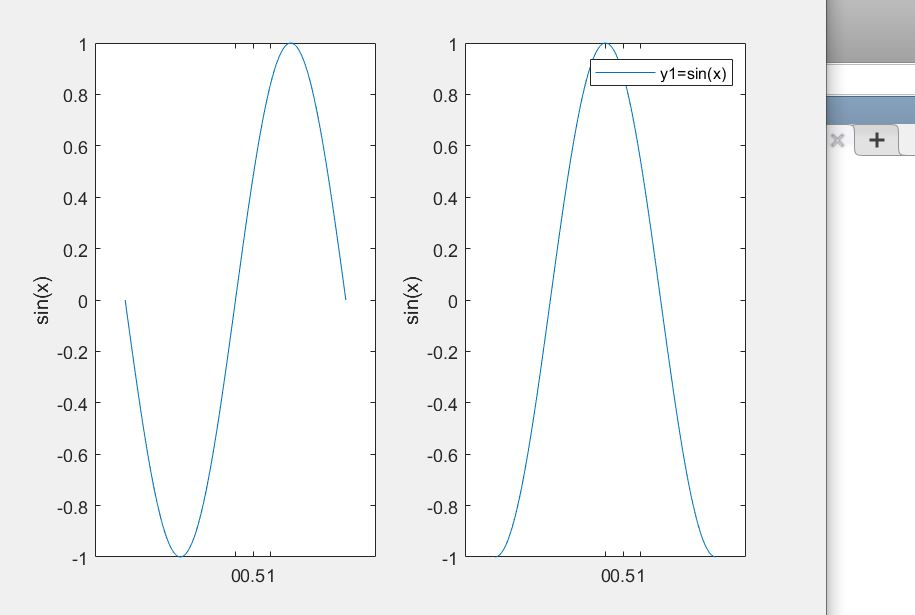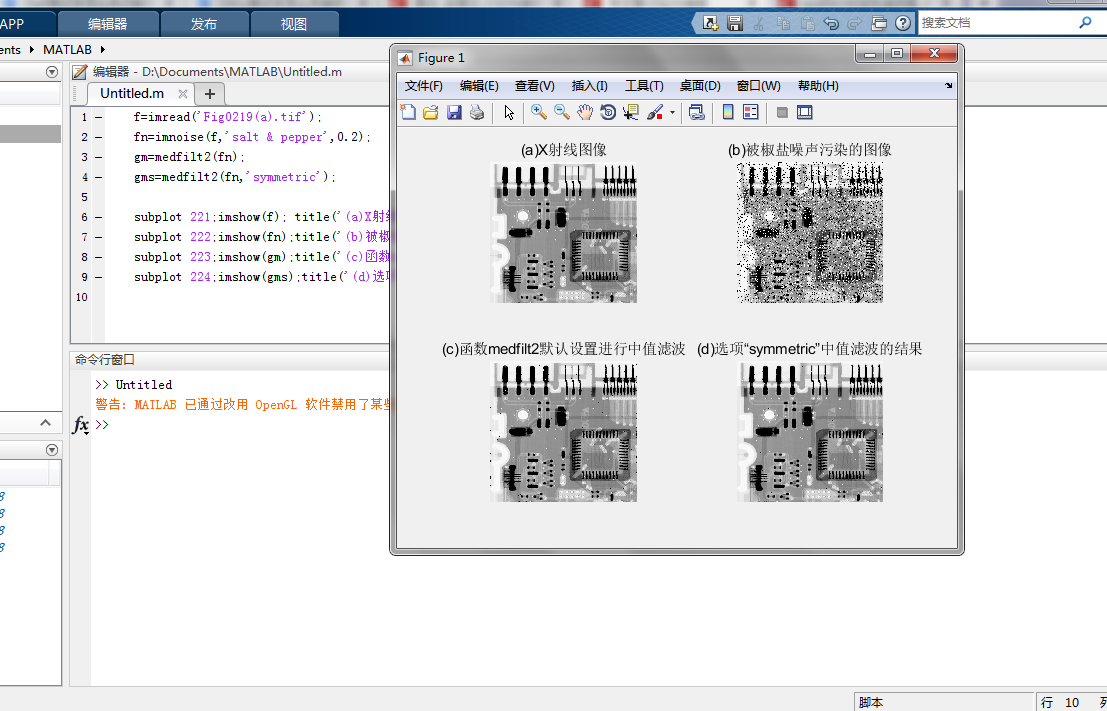• 怎样用MATLAB进行画图呢？...subplot(1,2,1) ：1,2表示把这两个图按照一行两列的方式排列，最后一1表示把这个图放在第一位置上 下面是我写的程序，供大家参考 clear all clc x=linspace(-pi,pi,100); ...

怎样用MATLAB进行画图呢？
例如我要画y1=sin(x); y2=cos(x);并把他们放在两个不同的框并列起来，MATLAB提供了函数plot(x,y1)：画图
subplot(1,2,1) ：1,2表示把这两个图按照一行两列的方式排列，最后一个1表示把这个图放在第一个位置上
下面是我写的程序，供大家参考

clear all
clc
x=linspace(-pi,pi,100); %把x分裂成100个点
y1=sin(x);
y2=cos(x);

subplot(1,2,1) %几行几列第几个图
plot(x,y1)
ylabel(‘sin(x)’)
set(gca,‘xtick’,[0 0.5 1]) %加上图线标注
subplot(1,2,2)
plot(x,y2)
legend(“y1=sin(x)”,“y2=cos(x)”) %为图像加上标题
xlabel(‘x’)
ylabel(‘sin(x)’) %在y坐标上增加批注
set(gca,‘xtick’,[0 0.5 1]) %加上图线标注
最后画出来这个样子：如果你想把两个图放在一个图中也很简单，直接plot(x,y1,x,y2)就可以了

展开全文MATLAB画图
• matlab怎么把两个图放在一起

千次阅读 2021-04-18 03:16:21
1.matlab怎么把两个图放在一起MATLAB中有hold on和hold off指令，在Command Window中使用可以实现同时让两个图出现在一起。hold on的意思是打印的图形会叠加在当前图形上。hold off的意思是打印图形前会消除当前图形...

1.matlab怎么把两个图放在一起

MATLAB中有hold on和hold off指令，在Command Window中使用可以实现同时让两个图出现在一起。

hold on的意思是打印的图形会叠加在当前图形上。

hold off的意思是打印图形前会消除当前图形，再打印新的图形。

具体可以在Command Window中输入help hold查询。

具体使用方法如下：

plot…… %打印第一个图

hold on

plot…… %打印第二个图，叠加到第一个图上去

hold off

2.matlab怎样将两张图放到一张图上

具体步骤如下所示：

1、打开电脑，进入电脑的主页面，打开软件，进入软件的主页，如下图所示。

2、进入软件之后，要把两张图放在一张图上，就会看到以下画面，可以使用hold on 语句，就如下图所示一样。

3、这样用上面的hold on 语句之后，这样画一个图形之后，继续画第二个，就会看到两张图在一个画面中了。

3.MATLAB中怎样将两张图叠放在一起

1.用hold on;hold off

例子

ezplot('sin')

hold on

ezplot('cos')

ezplot('tan')

ezplot('cot')

hold off

2.用subplot

例子

subplot(2,2,1)

ezplot('sin')

subplot(2,2,2)

ezplot('tan')

subplot(2,2,3)

ezplot('cos')

subplot(2,2,4)

ezplot('cot')

4.怎么把MATLAB生成的两个图合并在一起啊

可以使用subplot()函数完成。

使用方法：subplot(m,n,p)或者subplot(m n p)。

subplot是将多个图画到一个平面上的工具。其中，m表示是图排成m行，n表示图排成n列，也就是整个figure中有n个图是排成一行的，一共m行，如果m=2就是表示2行图。p表示图所在的位置，p=1表示从左到右从上到下的第一个位置。

在matlab的命令窗口中输入doc subplot或者help subplot即可获得该函数的帮助信息。

示例：

在MATLAB的命令窗口依次输入以下命令：(>>不用输入)

>>t=0:0.001:1;

>>y1=sin(10*t);

>>y2=sin(15*t);

>>subplot(211)

>>plot(t,y1)

>>subplot(212)

>>plot(t,y2)

5.如何在MATLAB里面将两个图画在一起

> data=[

74 781 5

1373 731 11

1321 1791 28

0 1787 4

1049 2127 12

1647 2728 6

2883 3617 15

2383 3692 7

2708 2295 22

2933 1767 7

];

T=data(:,1);

D=data(:,2);

K=data(:,3);

[X,Y,Z]=griddata(T,D,K,linspace(min(T),max(T))',linspace(min(D),max(D)),'v4')；%插值

contourf(X,Y,Z) %等高线图

hold on;plot(T,D,'-o');

展开全文• matlab中将图片放在一起显示 f=imread('Fig0219(a).tif'); fn=imnoise(f,'salt & pepper',0.2); gm=medfilt2(fn); gms=medfilt2(fn,'symmetric'); subplot 221;imshow(f); title('(a)X射线图像'); subplot ...

matlab中将几幅图片放在一起显示

fn=imnoise(f,'salt & pepper',0.2);
gm=medfilt2(fn);
gms=medfilt2(fn,'symmetric');

subplot 221;imshow(f); title('(a)X射线图像');
subplot 222;imshow(fn);title('(b)被椒盐噪声污染的图像');
subplot 223;imshow(gm);title('(c)函数medfilt2默认设置进行中值滤波');
subplot 224;imshow(gms);title('(d)选项“symmetric”中值滤波的结果');

运行截图:学到了一个很有用的技巧，有收获。

展开全文matlab 计算机视觉
• 该楼层疑似违规已被系统折叠 隐藏此楼查看此楼 A=[1790,3.9, 1800,5.3, 1810,7.2, 1820,9.6, 1830,12.9, 1840,17.1, ... plot(t,x,'o',tt,fun(a,tt)) format short g-- 怎么把上面两图像放在里面呢？谢谢了……

该楼层疑似违规已被系统折叠 隐藏此楼查看此楼

A=[1790,3.9,

1800,5.3,

1810,7.2,

1820,9.6,

1830,12.9,

1840,17.1,

1850,23.2,

1860,31.4,

1870,38.6,

1880,50.2,

1890,62.9,

1900,76.0,

1910,92.0,

1920,106.5,

1930,123.2,

1940,131.7,

1950,150.7,

1960,179.3,

1970,204.0,

1980,226.5,

1990,251.4]

t0=A(1,1);x0=A(1,2);

t=A(:,1);x=A(:,2);

fun=@(a,t) a(1)*x0*exp(a(2)*(t-t0))./(x0*a(3)*exp(a(2)*(t-t0))+a(1)-x0*a(3))

a=nlinfit(t,x,fun,[400 0.1 2]);

xm=a(1),r=a(2),c=a(3)

tt=1790:1990;

plot(t,x,'o',tt,fun(a,tt))

format short g--

A=[1790,3.9,

1800,5.3,

1810,7.2,

1820,9.6,

1830,12.9,

1840,17.1,

1850,23.2,

1860,31.4,

1870,38.6,

1880,50.2,

1890,62.9,

1900,76.0,

1910,92.0,

1920,106.5,

1930,123.2,

1940,131.7,

1950,150.7,

1960,179.3,

1970,204.0,

1980,226.5,

1990,251.4]

t0=A(1,1);x0=A(1,2);

t=A(:,1);x=A(:,2);

fun=@(a,t) a(1)*x0*exp(a(2)*(t-t0))./(x0*exp(a(2)*(t-t0))+a(1)-x0)

a=nlinfit(t,x,fun,[400 0.1]);

xm=a(1),r=a(2)

tt=1790:1990;

plot(t,x,'o',tt,fun(a,tt))

format short g--

怎么把上面两个图像放在一个里面呢？谢谢了……

展开全文• 有2张图，需要将其合在一起显示 clc clear close all path(path,'D:\new_matlab_Tool\matlab_tool_cwc\export_fig_v3') %figure('position',[50 50 800 800]) I = imread('1.png'); I1 = imread('2.png'); h1 = ...matlab
• 好像还是行，按你说的方法，把两程序放在一起，但还只是显示第二程序的，第一没有显示出来，这是程序原代码，再帮我看看吧%Generic Algorithm for function f(x1,x2) potimumclear all;close all;%...
• clc;closeall;%这句可以不要clearall;funtool;%这句可以在其他地方调用figs=findobj(0...%找到当前所有figure句柄%确定figure1和figure2，原理是figure3有不止一childreniflength(get(figs(1),'Children'))>1fi...
• 案例：之前跑过的程序 已经生成了多个matlab图，现在需要进行合并到一个图中。解决方案，利用图像句柄把figure图像中的参数读入到内存中，然后重新subplot绘制。程序如下：clc;clear;open(‘./test_1.fig’)figure_...
• MATLAB图像处理之直方匹配(图像合理增强)MATLAB图像处理之直方匹配(图像合理增强)先拿结果来看，第一幅是原 第二幅是直方均衡化后的图像增强 第三幅是直方匹配后的处理结果第二幅 第三幅来看 直接均衡化 ...
• 您可以使用Python，使用numpy和matplotlib模块生成这样一翻译（移位）图像堆栈。Pillow（另一Python模块）本身也可以做到这一点，但是我必须研究如何确保重叠像素的值被添加，而不是被覆盖。在下面是一numpy+...
• 0. 原由Matlab使用过程中，会遇到需要将多图形Figure文件合并成一文件的需求，便于在一坐标轴内对曲线进行合并的显示和比较。如下：分别使用P控制、PID控制、模糊PID控制、神经网络PID控制方法，得到了某...matlab simulink Figure
• 通常我们会将各个单独画出来看效果，但是写文章过程中，经常为了排版等问题，需要将几个图分别以子图的形式合为一个，这时候使用subplot，然后将各个重新在子图中画出，但是这种方法，我们需要将这些的画图...
• 1. 问题描述MATLAB第三方工具箱输出的图像，往往需要进一步处理，例如mark这两张图像，最好在MATLAB中合成到一figure中显示，以便对比模态位移的差别。2. 技术背景MATLAB中的图像，实际上是一对象集合，打开任意...
• MATLAB中，一般情况下生成的图例都是独占一行，代码如下 close all;clear all;clc; %第一组数据 a1 L1 = [1 2 3 4 5 6 ] W1 = [0.79 0.83 1.0 1.2 1.3 1.5 ] %第二组数据 b1 L2 = [ 1 2 3 4 5 6] W2 = [0.78...matlab
• load('mnist_uint8.mat');%加载mnist_uint8数据库 for i= 1:20 %获取20张图像保存在本地 img=reshape(test_x(i,:),[28 28])'; imwrite(img,strcat('D:\3rdlibrary\DeepLearnToolbox-master\data\',num2str(i),'....matlab 图片 montage
• matlab中可以利用三维数62616964757a686964616fe78988e69d8331333361323032据等值面绘制函数isosurface来绘制三维隐函数的图像三维隐函数可以描述，为方程 f(x,y,z)=0;如果我们定义函数v=f(x,y,z)在一定的下x，y，...
• 本文作者：合肥工业大学 管理学院 钱洋... 未经本人，允许禁止转载。如下所示为示例程序：figure; hold all; subplot(2,1,1); data = [2541, 1565, 1129, 962, 1175, 1112,728,640]; b = bar(data); set(gca,'XTickLmatlab
• 不同维度的箱线图画在一起123456789101112131415161718192021222324252627282930dataA = 10 *rand(100,1);% subplot(121);boxplot(dataA)dataB = 10 *rand(200,1) - 5*rand(200,1);% sub...
• matlab实现矩阵动态追加描述代码输出 描述 将for循环的每一循环结果都放在一起 代码 A = []; % 建立空矩阵 for i = 1:10 B = i^2; A = [A,B]; % 关键步骤 end 输出 ...matlab
• 1. 怎样用matlab把两figure中的叠加figure(1); x=-4:0.5:4; y=x [X,Y]=meshgrid(x,y); Z=X.^2+Y.^2; subplot(211) mesh(Z) h=mesh(Z) % figure(2); m=-4:0.5:4; n=m [M,N]=meshgrid(m,n); A=M.^2+N.^2; subplot...
• 为本人原创代码，用matlab实现多张图片同时在三维空间中显示，有例程，代码可直接运行。
• 共回答了13问题采纳率：92.3%clearR1=8*10^(-3);C1=1500;I=10;r=1.5;t=[0:100:400];A=-R1*r^4*(r-1)^2*(r^2+r+1)/((r^4+r^2+1)^2*(r+1));B=-t.*(r^4+r^2+1)*(r+1)/(R1*C1*(r^2+r+1)*(r^2+1));C=(R1*r^8*(r+1)+R1*r...
• MATLAB中把多张fig数据合并到同一张figure中 关键在于fig的名称需要有好的规律性，方便使用for循环 例如： 将文件夹中的4张fig放到一起，各的名称如下 代码如下 clc,clear all; %% 提取图片数据...matlab画图
• matlab最简单的代码因子 该项目的目标是创建一个MATLAB / C ++框架，以推断Forney风格的因子。 可以下载最新的软件包。...h(y,z)是几个变量的函数f(w,x,y,z)的因式分解。 表示这种分解的FFG如下...

matlab几个图放在一起matlab 订阅